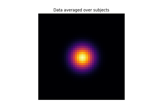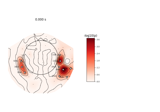# mne.stats.permutation_t_test¶

mne.stats.permutation_t_test(X, n_permutations=10000, tail=0, n_jobs=1, seed=None, verbose=None)[source]

One sample/paired sample permutation test based on a t-statistic.

This function can perform the test on one variable or simultaneously on multiple variables. When applying the test to multiple variables, the “tmax” method is used for adjusting the p-values of each variable for multiple comparisons. Like Bonferroni correction, this method adjusts p-values in a way that controls the family-wise error rate. However, the permutation method will be more powerful than Bonferroni correction when different variables in the test are correlated (see 1).

Parameters
X`array`, shape (n_samples, n_tests)

Samples (observations) by number of tests (variables).

n_permutations`int` | ‘all’

Number of permutations. If n_permutations is ‘all’ all possible permutations are tested. It’s the exact test, that can be untractable when the number of samples is big (e.g. > 20). If n_permutations >= 2**n_samples then the exact test is performed.

tail-1 or 0 or 1 (default = 0)

If tail is 1, the alternative hypothesis is that the mean of the data is greater than 0 (upper tailed test). If tail is 0, the alternative hypothesis is that the mean of the data is different than 0 (two tailed test). If tail is -1, the alternative hypothesis is that the mean of the data is less than 0 (lower tailed test).

n_jobs`int`

The number of jobs to run in parallel (default 1). Requires the joblib package.

seed`None` | `int` | instance of `RandomState`

If `seed` is an `int`, it will be used as a seed for `RandomState`. If `None`, the seed will be obtained from the operating system (see `RandomState` for details). Default is `None`.

verbose

If not None, override default verbose level (see `mne.verbose()` and Logging documentation for more). If used, it should be passed as a keyword-argument only.

Returns
T_obs`array` of shape [n_tests]

T-statistic observed for all variables.

p_values`array` of shape [n_tests]

P-values for all the tests (a.k.a. variables).

H0`array` of shape [n_permutations]

T-statistic obtained by permutations and t-max trick for multiple comparison.

Notes

If `n_permutations >= 2 ** (n_samples - (tail == 0))`, `n_permutations` and `seed` will be ignored since an exact test (full permutation test) will be performed.

References

1

Thomas E. Nichols and Andrew P. Holmes. Nonparametric permutation tests for functional neuroimaging: a primer with examples. Human Brain Mapping, 15(1):1–25, 2002. doi:10.1002/hbm.1058.

## Examples using `mne.stats.permutation_t_test`¶